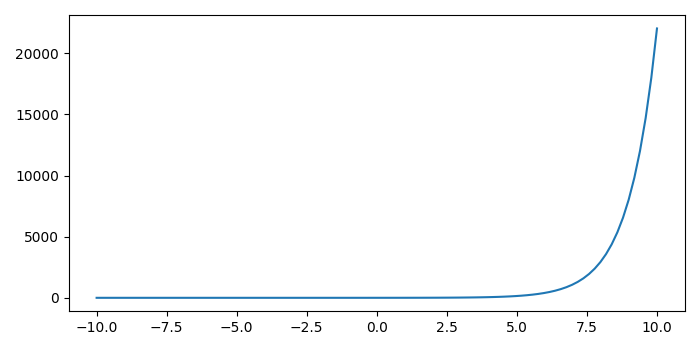# How get the (x,y) position pointing with mouse in an interactive plot (Python Matplotlib)?

MatplotlibPythonData Visualization

#### Python Data Science basics with Numpy, Pandas and Matplotlib

Most Popular

63 Lectures 6 hours

#### Data Visualization using MatPlotLib & Seaborn

11 Lectures 4 hours

#### MatPlotLib with Python

9 Lectures 2.5 hours

To get the (x, y) positions pointing with mouse in an interactive plot, we can take the following steps

## Steps

• Set the figure size and adjust the padding between and around the subplots.

• Create a new figure or activate an existing figure.

• Bind the function *mouse_event* to the event *button_press_event*.

• Create x and y data points using numpy.

• Plot the x and y data points using plot() method.

• To display the figure, use Show() method.

## Example

import numpy as np
from matplotlib import pyplot as plt

plt.rcParams["figure.figsize"] = [7.00, 3.50]
plt.rcParams["figure.autolayout"] = True

def mouse_event(event):
print('x: {} and y: {}'.format(event.xdata, event.ydata))

fig = plt.figure()
cid = fig.canvas.mpl_connect('button_press_event', mouse_event)

x = np.linspace(-10, 10, 100)
y = np.exp(x)

plt.plot(x, y)

plt.show()

## Output

It will produce the following output −Now, click anywhere on the plot and it will show the coordinates of the points on the console −

x: -3.633289020076159 and y: 7344.564590474489
x: 3.2193731551790172 and y: 3255.6463283494704
x: 8.680088326085489 and y: 802.2953710744596
x: 7.680741758860773 and y: 11269.926122114506
x: 0.6139338906288732 and y: 16503.741497634528